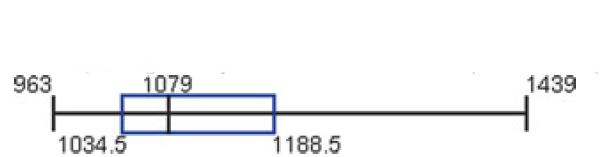×
Get Full Access to Elementary Statistics - 12 Edition - Chapter 3.4 - Problem 9re
Get Full Access to Elementary Statistics - 12 Edition - Chapter 3.4 - Problem 9re

×

# Interpreting a Boxplot Shown below is a boxplot of aISBN: 9780321836960 18

## Solution for problem 9RE Chapter 3.4

Elementary Statistics | 12th Edition

• Textbook Solutions
• 2901 Step-by-step solutions solved by professors and subject experts
• Get 24/7 help from StudySoup virtual teaching assistantsElementary Statistics | 12th Edition

4 5 1 401 Reviews
15
1
Problem 9RE

Interpreting a Boxplot Shown below is a boxplot of a sample of 20 brain volumes $$((\operatorname{cm} 3)$$. What do the numbers in the boxplot represent?Equation Transcription:Text Transcription:

( cm 3)

Step-by-Step Solution:
Step 1 of 3

Solution 9RE

From the given information, the given box plot isTherefore, the 5-number summary from the given box plot is minimum data value 963, first quartile value is 1034.5, second quartile value is 1079, third quartile value is 1188.5, and maximum value is 1439.

Step 2 of 3

Step 3 of 3

##### ISBN: 9780321836960

The answer to “?Interpreting a Boxplot Shown below is a boxplot of a sample of 20 brain volumes $$((\operatorname{cm} 3)$$. What do the numbers in the boxplot represent? Equation Transcription:Text Transcription:( cm 3)” is broken down into a number of easy to follow steps, and 30 words. Since the solution to 9RE from 3.4 chapter was answered, more than 475 students have viewed the full step-by-step answer. This textbook survival guide was created for the textbook: Elementary Statistics, edition: 12. This full solution covers the following key subjects: boxplot, below, brain, Interpreting, Numbers. This expansive textbook survival guide covers 121 chapters, and 3629 solutions. Elementary Statistics was written by and is associated to the ISBN: 9780321836960. The full step-by-step solution to problem: 9RE from chapter: 3.4 was answered by , our top Statistics solution expert on 03/15/17, 10:30PM.

## Discover and learn what students are asking

Calculus: Early Transcendental Functions : Differential Equations: Separation of Variables
?In Exercises 1-14, find the general solution of the differential equation. $$x^{2}+5 y \frac{d y}{d x}=0$$

Statistics: Informed Decisions Using Data : Testing the Significance of the Least-Squares Regression Model
?In Problems 5–10, use the results of Problems 7–12, respectively, from Section 4.2 to answer the following questions: (a) What are the estima

Unlock Textbook Solution